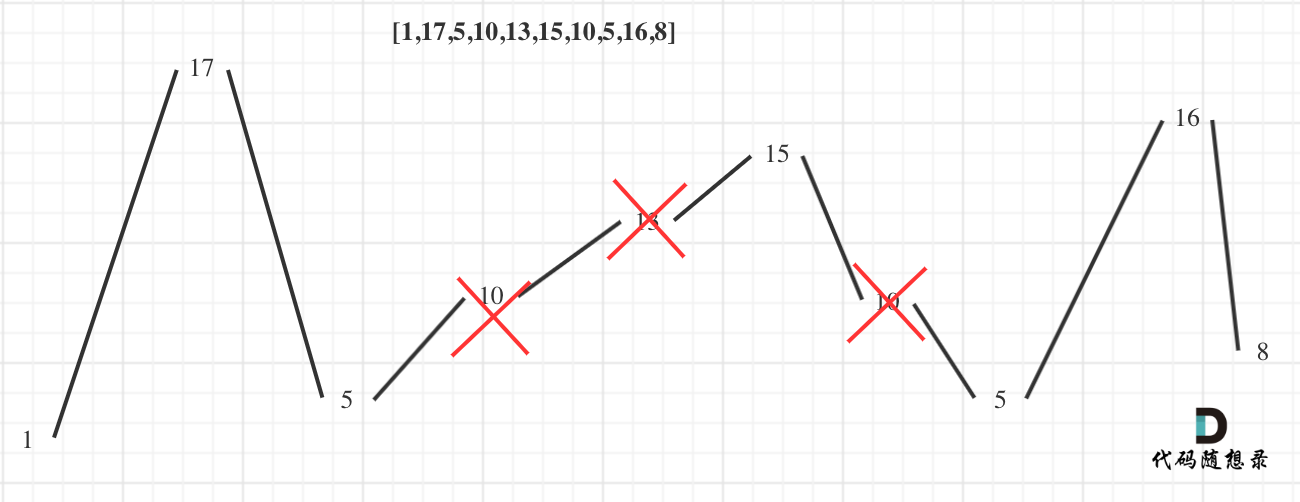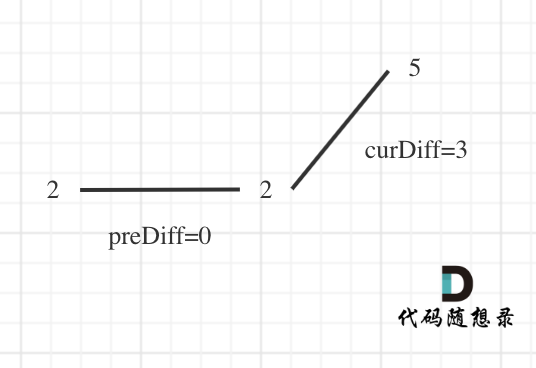# 376. 摆动序列

• 输入: [1,7,4,9,2,5]
• 输出: 6
• 解释: 整个序列均为摆动序列。

• 输入: [1,17,5,10,13,15,10,5,16,8]
• 输出: 7
• 解释: 这个序列包含几个长度为 7 摆动序列，其中一个可为[1,17,10,13,10,16,8]。

• 输入: [1,2,3,4,5,6,7,8,9]
• 输出: 2

# 思路1（贪心解法）（为方便表述，以下说的峰值都是指局部峰值）C++代码如下（和上图是对应的逻辑）：

class Solution {
public:
int wiggleMaxLength(vector<int>& nums) {
if (nums.size() <= 1) return nums.size();
int curDiff = 0; // 当前一对差值
int preDiff = 0; // 前一对差值
int result = 1;  // 记录峰值个数，序列默认序列最右边有一个峰值
for (int i = 0; i < nums.size() - 1; i++) {
curDiff = nums[i + 1] - nums[i];
// 出现峰值
if ((curDiff > 0 && preDiff <= 0) || (preDiff >= 0 && curDiff < 0)) {
result++;
preDiff = curDiff;
}
}
return result;
}
};
1
2
3
4
5
6
7
8
9
10
11
12
13
14
15
16
17
18
• 时间复杂度：\$O(n)\$
• 空间复杂度：\$O(1)\$

# 思路2（动态规划）

• 设dp状态dp[i]，表示考虑前i个数，第i个数作为山峰的摆动子序列的最长长度
• 设dp状态dp[i]，表示考虑前i个数，第i个数作为山谷的摆动子序列的最长长度

• dp[i] = max(dp[i], dp[j] + 1)，其中0 < j < inums[j] < nums[i]，表示将nums[i]接到前面某个山谷后面，作为山峰。
• dp[i] = max(dp[i], dp[j] + 1)，其中0 < j < inums[j] > nums[i]，表示将nums[i]接到前面某个山峰后面，作为山谷。

C++代码如下：

class Solution {
public:
int dp;
int wiggleMaxLength(vector<int>& nums) {
memset(dp, 0, sizeof dp);
dp = dp = 1;

for (int i = 1; i < nums.size(); ++i)
{
dp[i] = dp[i] = 1;

for (int j = 0; j < i; ++j)
{
if (nums[j] > nums[i]) dp[i] = max(dp[i], dp[j] + 1);
}

for (int j = 0; j < i; ++j)
{
if (nums[j] < nums[i]) dp[i] = max(dp[i], dp[j] + 1);
}
}
return max(dp[nums.size() - 1], dp[nums.size() - 1]);
}
};
1
2
3
4
5
6
7
8
9
10
11
12
13
14
15
16
17
18
19
20
21
22
23
24
• 时间复杂度：\$O(n^2)\$
• 空间复杂度：\$O(n)\$

• 每次更新dp[i]，则在tree1nums[i]位置值更新为dp[i]
• 每次更新dp[i]，则在tree2nums[i]位置值更新为dp[i]
• 则dp转移方程中就没有必要j从0遍历到i-1，可以直接在线段树中查询指定区间的值即可。

# 其他语言版本

# Java

class Solution {
public int wiggleMaxLength(int[] nums) {
if (nums == null || nums.length <= 1) {
return nums.length;
}
//当前差值
int curDiff = 0;
//上一个差值
int preDiff = 0;
int count = 1;
for (int i = 1; i < nums.length; i++) {
//得到当前差值
curDiff = nums[i] - nums[i - 1];
//如果当前差值和上一个差值为一正一负
//等于0的情况表示初始时的preDiff
if ((curDiff > 0 && preDiff <= 0) || (curDiff < 0 && preDiff >= 0)) {
count++;
preDiff = curDiff;
}
}
return count;
}
}
1
2
3
4
5
6
7
8
9
10
11
12
13
14
15
16
17
18
19
20
21
22
23
// DP
class Solution {
public int wiggleMaxLength(int[] nums) {
// 0 i 作为波峰的最大长度
// 1 i 作为波谷的最大长度
int dp[][] = new int[nums.length];

dp = dp = 1;
for (int i = 1; i < nums.length; i++){
//i 自己可以成为波峰或者波谷
dp[i] = dp[i] = 1;

for (int j = 0; j < i; j++){
if (nums[j] > nums[i]){
// i 是波谷
dp[i] = Math.max(dp[i], dp[j] + 1);
}
if (nums[j] < nums[i]){
// i 是波峰
dp[i] = Math.max(dp[i], dp[j] + 1);
}
}
}

return Math.max(dp[nums.length - 1], dp[nums.length - 1]);
}
}
1
2
3
4
5
6
7
8
9
10
11
12
13
14
15
16
17
18
19
20
21
22
23
24
25
26
27

# Python

class Solution:
def wiggleMaxLength(self, nums: List[int]) -> int:
preC,curC,res = 0,0,1  #题目里nums长度大于等于1，当长度为1时，其实到不了for循环里去，所以不用考虑nums长度
for i in range(len(nums) - 1):
curC = nums[i + 1] - nums[i]
if curC * preC <= 0 and curC !=0:  #差值为0时，不算摆动
res += 1
preC = curC  #如果当前差值和上一个差值为一正一负时，才需要用当前差值替代上一个差值
return res
1
2
3
4
5
6
7
8
9

# Go

func wiggleMaxLength(nums []int) int {
var count,preDiff,curDiff  int
count=1
if len(nums)<2{
return count
}
for i:=0;i<len(nums)-1;i++{
curDiff=nums[i+1]-nums[i]
//如果有正有负则更新下标值||或者只有前一个元素为0（针对两个不等元素的序列也视作摆动序列，且摆动长度为2）
if (curDiff > 0 && preDiff <= 0) || (preDiff >= 0 && curDiff < 0){
preDiff=curDiff
count++
}
}
return count
}
1
2
3
4
5
6
7
8
9
10
11
12
13
14
15
16

# Javascript

var wiggleMaxLength = function(nums) {
if(nums.length <= 1) return nums.length
let result = 1
let preDiff = 0
let curDiff = 0
for(let i = 0; i < nums.length - 1; i++) {
curDiff = nums[i + 1] - nums[i]
if((curDiff > 0 && preDiff <= 0) || (curDiff < 0 && preDiff >= 0)) {
result++
preDiff = curDiff
}
}
return result
};
1
2
3
4
5
6
7
8
9
10
11
12
13
14

var wiggleMaxLength = function(nums) {
if (nums.length === 1) return 1;
// 考虑前i个数，当第i个值作为峰谷时的情况（则第i-1是峰顶）
let down = 1;
// 考虑前i个数，当第i个值作为峰顶时的情况（则第i-1是峰谷）
let up = 1;
for (let i = 1; i < nums.length; i++) {
if (nums[i] < nums[i - 1]) {
down = Math.max(up + 1, down);
}
if (nums[i] > nums[i - 1]) {
up = Math.max(down + 1, up)
}
}
return Math.max(down, up);
};
1
2
3
4
5
6
7
8
9
10
11
12
13
14
15
16@2021-2022 代码随想录 版权所有 粤ICP备19156078号-3